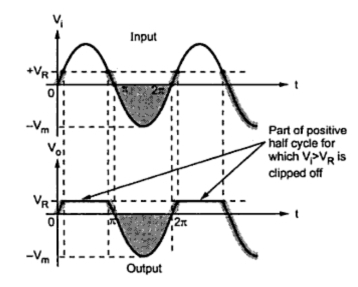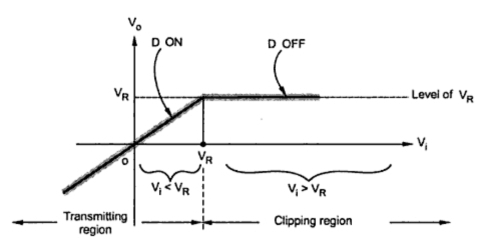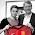Clipping above Reference Voltage VR

Series Clippers : Part4
The output of the clipper can be adjusted as per the requirement by adding an additional voltage source in series with the load resistance as shown in the Fig. 1.
The diode D is an ideal diode and hence there is no drop across it when it is forward biased. Thus when forward biased, it acts as a short circuit while when reverse biased it acts as open circuit.
Operation :
When Vi is less than VR, the diode becomes forward biased and the circuit can be reduced as shown in the Fig. 2(a). In this case the output voltage Vo is equal to input voltage Vi. When Vi is greater than VR, the diode is reverse biased and circuit gets reduced to, as shown in the Fig. 2(b). No current can flow in the circuit as circuit is open and hence output voltage Vo is equal to VR.Fig.  2
The input and output waveforms for such a clipper are shown in the Fig. 3.Fig. 3  Waveforms for clipping above VR
Note : It can be seen that the portion of the input waveform above VR gets clipped off. The level of  VR can be adjusted as per the requirement by selecting proper battery voltage.
Mathematically this can be expressed as,
This mathematical representation helps us to sketch the transfer characteristics of the clipper circuit. The transfer characteristics is the graph of output voltage Vo against input voltage Vi. The above equations are called the transfer characteristics is shown in the Fig. 4.Fig. 4  Transfer characteristics

For the portion till Vi < VR, the graph is straight line. This region is called the transmission region as it transmits Vi at the output as it is. While the portion for Vi >VR, the output is constant. This region is called clipping region.

1.